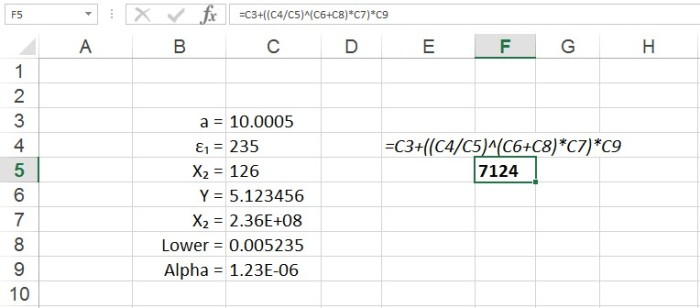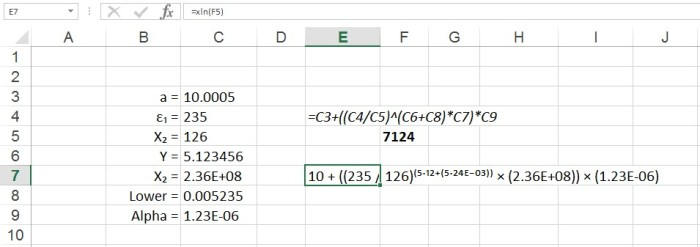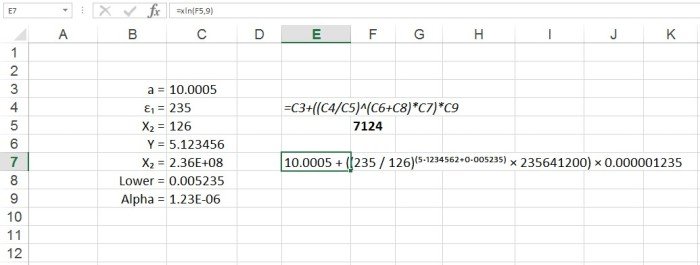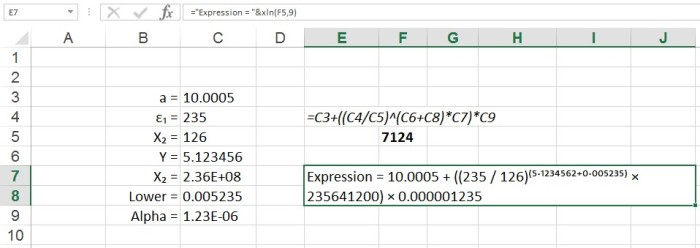# The XLN Function

## Function for displaying math formula with component values – xln

=xln(CELLREF, INTEGER)
This function displays the numerical version of a mathematical formula defined in another cell. It updates automatically with a change in the values in the formula or a change in the formula.

Example

A function has been created in Cell F5 that references the set of variables to left.The formula has been shown in cell E4 for clarity. The xln function can be used to show the values in the formula – it has been used in cell E7 in the figure belowThe xln function has been used without the INTEGER parameter and so has defaulted to its adaptive formatting. The adaptive formatting works in the following way:

Each value in the formula is parsed in the following way:

if Value > 10000000 the number is expressed in Scientific notation
If 10000000 > Value > 100 the number in expressed in standard notation to zero decimal places
If 100 > Value > .001 the number in expressed in standard notation to 3 significant figures
if Value < .001 the number is expressed in Scientific notation

The xln function can be modified using the optional integer parameter that sets the number of decimal places used for every value in the formula. In this mode, all values are expressed in standard notation. In the modified example below, the optional parameter has been set to 9:The xln function can be combined with other functions in the same cell to modify the way the formula is displayed. As the xln function returns the formula as a value in a cell, the formula will react in the same way as any other cell content or value.

In the modified example below, the output has been modified with some preceding text and the cells have been merged and the text wrapped: